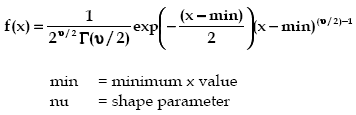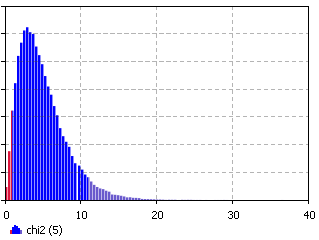AnyLogic

# chi2The Chi Squared is a continuous distribution bounded on the lower side. Note that the Chi Squared distribution is a subset of the Gamma distribution with beta=2 and alpha=nμ/2. Like the Gamma distribution, it has three distinct regions. For nμ = 2, the Chi Squared distribution reduces to the Exponential distribution, starting at a finite value at minimum x and decreasing monotonically thereafter. For nμ < 2, the Chi Squared distribution tends to infinity at minimum x and decreases monotonically for increasing x. For nμ > 2, the Chi Squared distribution is 0 at minimum x, peaks at a value that depends on nµ, decreasing monotonically thereafter.

Because the Chi Squared distribution does not have a scaling parameter, its utilization is somewhat limited. Frequently, this distribution will try to represent data with a clustered distribution with nµ less than 2. However, it can be viewed as the distribution of the sum of squares of independent unit normal variables with nµ degrees of freedom and is used in many statistical tests.

Examples of each of the regions of the Chi Squared distribution are shown above. Note that the peak of the distribution moves away from the minimum value for increasing nu, but with a much broader distribution.

#### Example (both high and low percentiles = 5)### chi2(double nu, double min)

Description
Generates a sample of the Chi Squared distribution.
Parameters
Name Type of value Description
nu double The shape parameter.
min double The minimum x value.
Result
Type Description
double the generated sample

### chi2(double nu)

Description
Generates a sample of the Chi Squared distribution with min set to 0. Is equivalent to chi2(nu, 0).
Parameters
Name Type of value Description
nu double The shape parameter.
Result
Type Description
double The generated sample.

### chi2(double nu, double min, java.util.Random r)

Description
Generates a sample of the Chi Squared distribution using the specified random number generator.
Parameters
Name Type of value Description
nu double The shape parameter.
min double The minimum x value.
r java.util.Random The random number generator.
Result
Type Description
double The generated sample.

This document includes content from the “Stat::Fit User’s Manual”. Copyright 2016 Geer Mountain Software Corp.